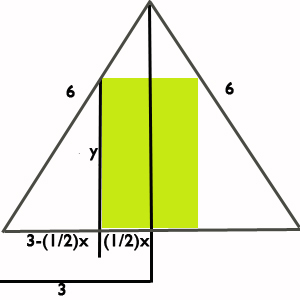# Calculus Problem of the Week September 9, 2011

Sometimes easy and sometimes hard, our calculus problem of the week could come from any calculus topic. If you really want to get better at calculus, following these problems is a great way to make yourself practice! Past calculus problems of the week.

This week’s problem:
(click “see the solution” at the bottom of post to, well, see the solution.)

Suppose you have an equilateral triangle whose sides are each 6 inches. Let’s say you were to draw a rectangle inside of this triangle such that the base of the rectangle was on the base of the triangle. What is the maximum area your rectangle can have?

See the solution.

The first thing you have to do here is a little bit of a “leap of faith”. In other words, we have to decide where in the triangle the rectangle would have to be in order to get the most area. I think it is obvious (there is the leap of faith!) that it would have to be in the middle. Feel free to argue with me in the comments about this but it is certainly true. Here is my picture:I have decided to call the bottom part of the rectangle$x$ and the longer part$y$. If we want to maximize area, then we want to maximize$A=xy$. The problem now becomes: “How do we get this into one variable?”. Once I do this, then I can easily take the derivative and find the maximum value of this area function!In the picture above, I started thinking about what information I could get out of our original picture. I know each side is 6 inches in length. So, if I drop down from the top of the triangle, I should have length 3 on each side. Also, since the rectangle is in the center, I will have half that length on one side of the line and half on the other. The remaining length would then be$3-\frac{1}{2}x$. My goal is to write$y$ in terms of$x$. We are closer to this than you think!Take a look at the highlighted triangle. That triangle was there the whole time I just added a little information to it. The big piece of information I added is the fact that the angle to the left is 60 degrees. I know this because for any equilateral triangle (triangle with sides that are all the same), the angles are 60 degrees.

The next thing to notice is that the highlighted triangle is a RIGHT triangle. This means that I can use a trig function to determine sides. In fact, I can say that:$\tan(60 deg)=\dfrac{y}{3-\frac{1}{2}x}$.
Remember$\tan(\theta)=\dfrac{opp}{adj}$

Since$\tan(60 deg)=\sqrt{3}$, I can solve for$y$ and get:$y=\sqrt{3}(3-\frac{1}{2}x)$

Plugging this back into area:$A=xy=x(\sqrt{3}(3-\dfrac{1}{2}x)=3x\sqrt{3}-\dfrac{\sqrt{3}}{2}x^2$

Finally, to find the maximum area I will take the derivative of this function, find when its derivative is zero, and finally plug that value back into the area function.
Finding the derivative:$A'=3\sqrt{3}-\sqrt{3}x$

Finding when the derivative is zero:$3\sqrt{3}-x\sqrt{3}=0$$-x\sqrt{3}=-3\sqrt{3}$$x=3$

Using the value of x to find the maximum area:$A=3x\sqrt{3}-\dfrac{\sqrt{3}}{2}x^2$$A=3(3)\sqrt{3}-\dfrac{\sqrt{3}}{2}(3)^2 = \dfrac{9\sqrt{3}}{2} = 7.79$ square inches.